# Variation of a functional

(diff) ← Older revision | Latest revision (diff) | Newer revision → (diff)

first variation

A generalization of the concept of the differential of a function of one variable. It is the principal linear part of the increment of the functional in a certain direction; it is employed in the theory of extremal problems to obtain necessary and sufficient conditions for an extremum. This was the meaning of the term "variation of a functional" imparted to it as early as 1760 by J.L. Lagrange . He considered, in particular, the functionals of the classical calculus of variations of the form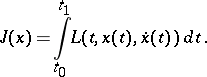(1)

If a given functionis replaced by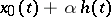and the latter is substituted in the expression for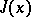, one obtains, assuming that the integrandis continuously differentiable, the following equation: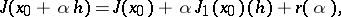(2)

where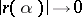as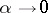. The function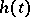is often referred to as the variation of the function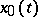, and is sometimes denoted by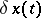. The expression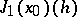, which is a functional with respect to the variation, is said to be the first variation of the functionaland is denoted by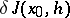. As applied to the functional (1), the expression for the first variation has the form(3)

where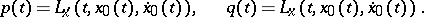A necessary condition for an extremum of the functionalis that the first variation vanishes for all. In the case of the functional (1), a consequence of this necessary condition and the fundamental lemma of variational calculus (cf. du Bois-Reymond lemma) is the Euler equation: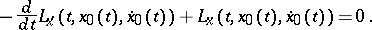A method similar to (2) is also used to determine variations of higher orders (see, for example, Second variation of a functional).

The general definition of the first variation in infinite-dimensional analysis was given by R. Gâteaux in 1913 (see Gâteaux variation). It is essentially identical with the definition of Lagrange. The first variation of a functional is a homogeneous, but not necessarily linear functional. The usual name under the additional assumption that the expressionis linear and continuous with respect tois Gâteaux derivative. Terms such as "Gâteaux variation" , "Gâteaux derivative" , "Gâteaux differential" are more frequently employed than the term "variation of a functional" , which is reserved for the functionals of the classical variational calculus .

How to Cite This Entry:
Variation of a functional. Encyclopedia of Mathematics. URL: http://encyclopediaofmath.org/index.php?title=Variation_of_a_functional&oldid=13685
This article was adapted from an original article by V.M. Tikhomirov (originator), which appeared in Encyclopedia of Mathematics - ISBN 1402006098. See original article